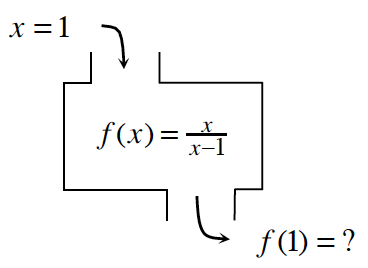### Home > CC4 > Chapter 2 > Lesson 2.1.2 > Problem2-21

2-21.

Calculate the output for the function with the given input. If there is no possible output for the given input, explain why not. Homework Help ✎

Substitute $1$ in for $x$ and evaluate the function.

$f(1)=\frac{1}{1-1}$

$f(1)=\frac{1}{0}$

What does it mean when there is a zero in the denominator?